## Arbitrary Computation on the GPU with ❤WebGL

### Seth Samuel (NY ↝ PDX ↝ Elsewhere ↝ PDX ↝ NYC)@sethfsamuelsethsamuel

# What is WebGL?``gl_FragColor = vec4(vCoord.s, vCoord.t, 0, 1.0);``

### Edge Finder

`````` void main(void) {
vec4 c = texture2D(uSampler, vCoord);
vec4 cLeft = texture2D(
uSampler, vCoord - vec2(-0.005,0.0)
);
if(distance(cLeft.rgb, c.rgb) > uThreshold){
gl_FragColor = vec4(1.0,0.0,1.0,1.0);
}else{
gl_FragColor = vec4(0.0,0.0,0.0,1.0);
}
}``````

### Particles

``````gl_Position = vec4(
sin(vPosition.x+uThreshold*6.28*vPosition.z*vPosition.z),
cos(vPosition.y+uThreshold*6.28*vPosition.z),
vPosition.z,
1.0
);``````

# How could we get data out of WebGL?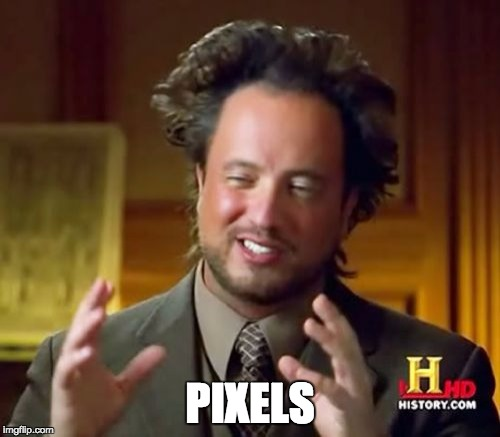# ``canvas.getImageData``

## Implementation Challenges# The high cost of serialization

### Matrix Muliplication

``````float sum = 0.0;
for(int i = 0; i < size; i++) {
vec4 cLeft  = texture2D(uSamplerLeft, vec2(i, vCoord.t));
vec4 cRight = texture2D(uSamplerRight, vec2(vCoord.s, i));
sum = sum + (cLeft * cRight);
}``````

# O(n) < O(n3)

## n ∈ [0, 5]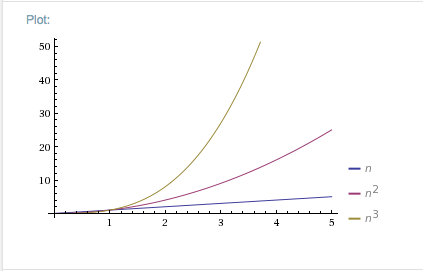## n ∈ [0, 10]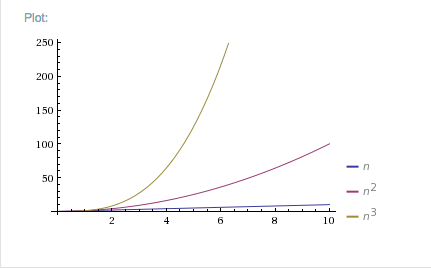## n ∈ [0, 100]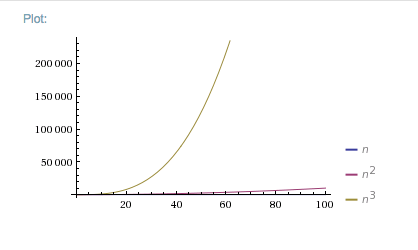## n ∈ [0, 1024]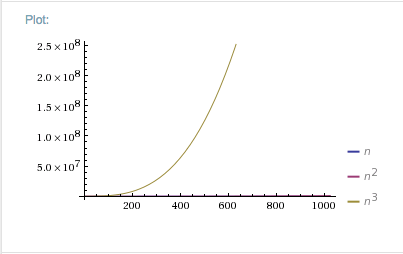# Actual Applications

## Implementation Blockers# What do we need to make it work?@sethfsamuelsethsamuel